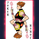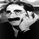10310 views
This calculates normal distance of price from VWAP . This is a mean reverting idea (something like ZScore), but using both "volume" and "close".

Useful for finding OB/OS areas and potential turning points.

Complete list of my indicators:
```//
// @author LazyBear
// List of all my indicators: https://www.tradingview.com/v/4IneGo8h/
//
study("Z distance from VWAP [LazyBear]", shorttitle="ZVWAP_LB")
length=input(20)

calc_zvwap(pds) =>
mean = sum(volume*close,pds)/sum(volume,pds)
vwapsd = sqrt(sma(pow(close-mean, 2), pds) )
(close-mean)/vwapsd

plot(0)
upperTop=input(2.5)
upperBottom=input(2.0)
lowerTop=input(-2.5)
lowerBottom=input(-2.0)

plot(1, style=3, color=gray), plot(-1, style=3, color=gray)
ul1=plot(upperTop, "OB High")
ul2=plot(upperBottom, "OB Low")
fill(ul1,ul2, color=red)
ll1=plot(lowerTop, "OS High")
ll2=plot(lowerBottom, "OS Low")
fill(ll1,ll2, color=green)
plot(calc_zvwap(length),title="ZVWAP",color=maroon, linewidth=2)

```
List of my free indicators: http://bit.ly/1LQaPK8
List of my indicators at Appstore: http://blog.tradingview.com/?p=970